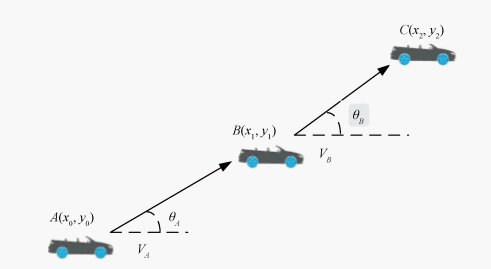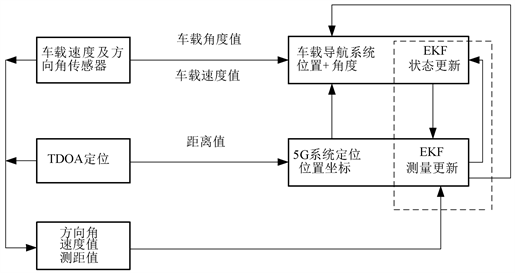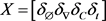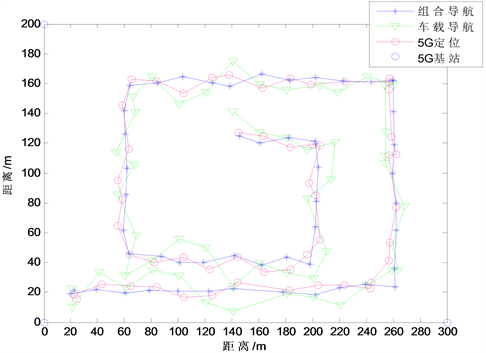# 基于5G与车载传感器的车辆组合导航系统研究Research on Vehicle Integrated Navigation System Based on 5G-Vehicle Sensors

DOI: 10.12677/CSA.2020.109170, PDF, HTML, XML, 下载: 246  浏览: 491  科研立项经费支持

Abstract: Vehicle positioning is an important part of the Internet of Vehicles technology. The vehicle-mounted global navigation satellite system is characterized with poor positioning accuracy and even inability to locate in the environment with complex multipath interference such as dense urban high-rise areas. With the rapid development of 5G wireless communication, it is of great significance to use combined positioning to improve vehicle positioning accuracy. In this paper, by studying the speed and yaw angle information collected by the sensors during vehicle operation, combined with the 5G communication system, a 5G-based vehicle-mounted integrated navigation system is proposed to improve the positioning accuracy. The simulation results show that the vehicle positioning accuracy can be effectively improved by the integrated navigation system in indoor or indoor-outdoor boundary lack of GNSS signal.

1. 引言

2. 车载自主导航系统

$\left[\begin{array}{c}{x}_{1}\\ {y}_{1}\end{array}\right]=\left[\begin{array}{c}{x}_{0}\\ {y}_{0}\end{array}\right]+\left[\begin{array}{c}{v}_{A}×T×{\theta }_{A}\\ {v}_{A}×T×{\theta }_{A}\end{array}\right]$ (1)Figure 1. Vehicle autonomous navigation calculation

$\left\{\begin{array}{l}{x}_{n}={x}_{0}+{\sum }_{i=1}^{n-1}{v}_{i}\mathrm{cos}{\theta }_{i}\\ {y}_{n}={y}_{0}+{\sum }_{i=0}^{n-1}{v}_{i}\mathrm{sin}{\theta }_{i}\end{array}$ (2)

3. 基于5G-车载传感器的组合导航系统

3.1. 5G系统定位原理

5G时代即将到来，5G衍生出的大规模MIMO、超密集网络、毫米波及D2D等关键技术将被广泛推广应用。毫米波具有大带宽，定位所需参数如到达时间(Time of Arrival, TOA)等的估计误差的克拉美罗下届小，同时抗干扰能力强。TOA定位依据测量接收信号在基站和移动台之间的到达时间，然后转换为距离来进行定位 。TOA需要移动台和基站之间严格同步，这将严重影响定位精度。基于信号达到时间差(Time Difference of Arrival, TDOA)通过检测信号到达基站的传播时间差，降低了算法对时间同步要求。

5G通信中，通过TDOA不仅能够提高测量的精度，还能够提高多径测量的分辨率。TDOA定位模型中，设A为待估计的移动台位置坐标 $\left(x,y\right)$，第i个基站 的坐标为别是BSi $\left({x}_{i},{y}_{i}\right)$ ，A点到达各个基站BSi的距离分别是 ${d}_{i}\left(i\in \left(1,2,\cdots ,N\right)\right)$，可通过如下公式计算得出 ：

${d}_{i}=\sqrt{{\left({x}_{i}-x\right)}^{2}+{\left({y}_{i}-y\right)}^{2}}$ (3)

TDOA通过计算两基站到移动台的时间差，从而计算出两基站到移动台的距离差，基站BS1和到其他基站BSi (i ≠ 1)的距离差可以由下面的公式求得：

${d}_{i,1}={d}_{i}-{d}_{1}=\sqrt{{\left({x}_{i}-x\right)}^{2}+{\left({y}_{i}-y\right)}^{2}}-\sqrt{{\left({x}_{1}-x\right)}^{2}+{\left({y}_{1}-y\right)}^{2}}$ (4)

3.2. 组合导航系统Figure 2. Diagram: integrated navigation system

$\left\{\begin{array}{l}{X}_{k+1}={F}_{k}\left({X}_{k},k\right)+{W}_{k}\\ {Y}_{k}={H}_{k}{X}_{k}+{V}_{k}\end{array}$ (5)

${F}_{k}={\frac{\partial F}{\partial X}|}_{X={\stackrel{^}{X}}_{k}^{-}},\text{\hspace{0.17em}}\text{\hspace{0.17em}}{H}_{k}={\frac{\partial H}{\partial X}|}_{X={\stackrel{^}{X}}_{k}^{-}}$ (6)(7)

$Y=\left[{\delta }_{\theta }{\delta }_{v}{\delta }_{x}{\delta }_{y}\right]$ (8)

4. 系统仿真与结果分析Figure 3. Trajectory simulationFigure 4. Error result comparisonTable 1. System resulting data of positioning error

5. 总结

  邱佳慧, 陈祎, 刘琪. 车联网标准化及其演进策略[J]. 移动通信, 2018, 42(4): 41-47.  许扬胤, 杨元喜, 何海波, 等. 北斗全球卫星导航系统试验卫星测距信号质量分析[J]. 武汉大学学报(信息科学版), 2018, 43(8): 1214-1221.  Wang, J., Ni, D. and Li, K. (2014) RFID-Based Vehicle Positioning and Its Applications in Connected Vehicles. Sensors, 14, 4225-4238. https://doi.org/10.3390/s140304225  刘雪梅. 基于第三代移动通信的蜂窝网无线定位技术的研究[D]: [硕士学位论文]. 哈尔滨: 哈尔滨工程大学, 2004.  程飞, 章平, 陈新泉, 等. 5G 移动通信系统中协作定位技术展望[J]. 天津理工大学学报, 2020, 36(2): 45-511.  Abu-Shaban, Z., Zhou, X., Abhayapala, T., Wymeersch, H., et al. (2018) Error Bounds for Uplink and Downlink 3D Localization in 5G Milli-meter Wave System. IEEE Transactions on Wireless Communications, 17, 4939-4954. https://doi.org/10.1109/TWC.2018.2832134  彭友志, 田野, 张炜程, 等. 5G/GNSS融合系统定位精度仿真分析[J]. 厦门大学学报(自然科学版), 2020, 59(1): 101-107.  李文娟. 车联网中车辆定位及其优化技术研究[D]: [硕士学位论文]. 南京: 南京邮电大学, 2013.  Garcia, N.,Wymeersch, H., Larsson, E.G., et al. (2017) Direct Localization for Massive MIMO. IEEE Transactions on Signal Processing, 65, 2475-2487. https://doi.org/10.1109/TSP.2017.2666779  曾焱垚, 赵久奋, 朱昱. 基于TDOA测量的协同导航建模与仿真[J]. 科学技术与工程, 2013, 13(9): 2513-2516.  黄金鹏, 尚俊娜, 岳克强, 等. 惯导系统辅助CSS的室内定位方法[J]. 传感技术学报, 2018, 31(3): 386-393.